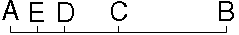Name: Richard Who is asking: Student Level: All Question:In order to transverse from point A to point B one must first cross the halfway point (C). Additionally....One must also pass another halfway point labeled (D) in order to get to the halfway point of (C). There is also point (E) which is the halfway point between A and D. We have to assume that there are an infinite amount of halfway points points between (A) and (B). My understanding of infinity is something that goes on forever. But how can one expect to traverse over infinity in a finite amount of time? Will we never reach (B)? Hi Richard, It is true that this LIST is infinite. Any effort to write it all down, with a fixed time for each recording of a position will take for ever.    The usual way this example is given is in Zeno's paradox, where you are going FROM B to A, with the same sequence of half-way points (traversed in order).    The secret to getting to A in a finite time is that each half-way step does NOT take the same time - the time per half-step is also going down (by 1/2). So the TIME to pass from B to A runs like: 1 + (1/2) + (1/2)2 + (1/2)2, one time step to get from B to C, 1/2 time step to get from C to D, (1/2)2 time steps to get from D to E... In fact the positions along the line correspond to the total time taken to get from B to the current position. The total time to get from B to A is 2!    We don't actually take specific steps for each of the 'half-ways'. Our last finite step will get us to the end, past an infinite number of points. Cheers, Walter Go to Math Central To return to the previous page use your browser's back button.